# Adding dogs to ggplot2 with ggdogs

R CODER

## Available dogs

The `ggdogs` package allows adding dog images instead of points in ggplot2 charts when using the `geom_dog` function instead of `geom_point`.

There are 15 different dogs available at this moment, named `"doge"` (default), `"doge_strong"`, `"chihuahua"`, `"eyes"`, `"gabe"`, `"glasses"`, `"tail"`, `"surprised"`, `"thisisfine"`, `"hearing"`, `"pug"`, `"ears"`, `"husky"`, `"husky_2"` and `"chilaquil"`. You can pass any of the previous strings to the function to use the corresponding dog.

The following figure shows all the available images:

``````# install.packages("remotes")
# remotes::install_github("R-CoderDotCom/ggdogs@main")
library(ggdogs)
# install.packages("ggplot2")
library(ggplot2)

grid <- expand.grid(1:5, 3:1)

df <- data.frame(x = grid[, 1],
y = grid[, 2],
image = c("doge", "doge_strong", "chihuahua",
"eyes", "gabe", "glasses",
"tail", "surprised", "thisisfine",
"hearing", "pug", "ears",
"husky", "husky_2", "chilaquil"))

ggplot(df) +
geom_dog(aes(x, y, dog = image), size = 5) +
geom_text(aes(x, y - 0.5, label = image), size = 2.5) +
xlim(c(0.25, 5.5)) +
ylim(c(0.25, 3.5))``````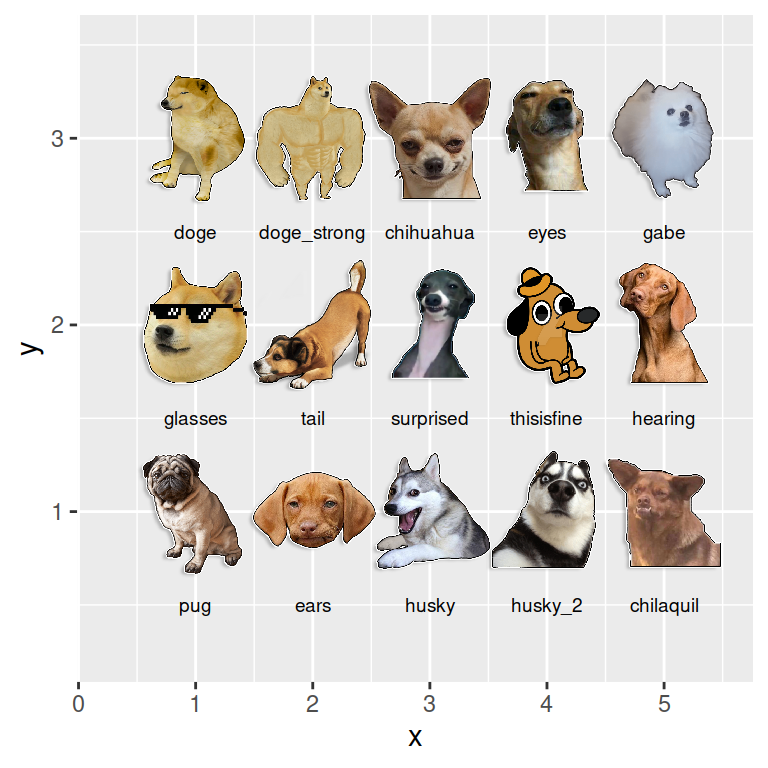## Creating a dog plot with `geom_dog`

Given a data frame you can use the `geom_dog` function the same way as `geom_point`. The main difference is that you will see Doge dogs instead of points by default. Note that you can set the size of the dogs with the `size` argument of the function.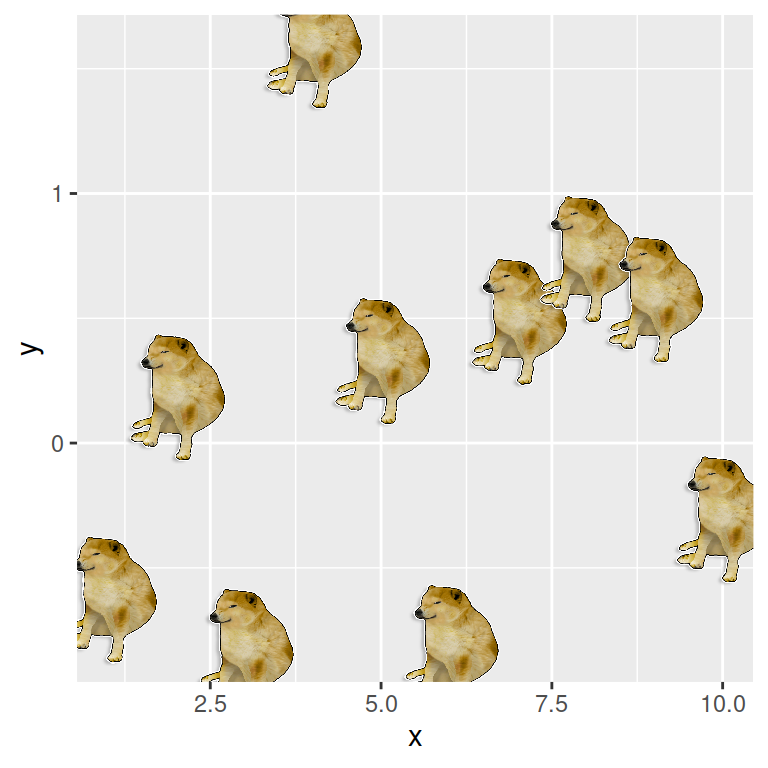``````# install.packages("remotes")
# remotes::install_github("R-CoderDotCom/ggdogs@main")
library(ggdogs)
# install.packages("ggplot2")
library(ggplot2)

# Sample data
set.seed(1)
df <- data.frame(x = 1:10, y = rnorm(10))

# Plot
ggplot(df, aes(x = x, y = y)) +
geom_dog(size = 5)``````

You can also combine a regular plot with `geom_dogs`. In the following example we are creating a scatter plot with the image of a dog plus a text.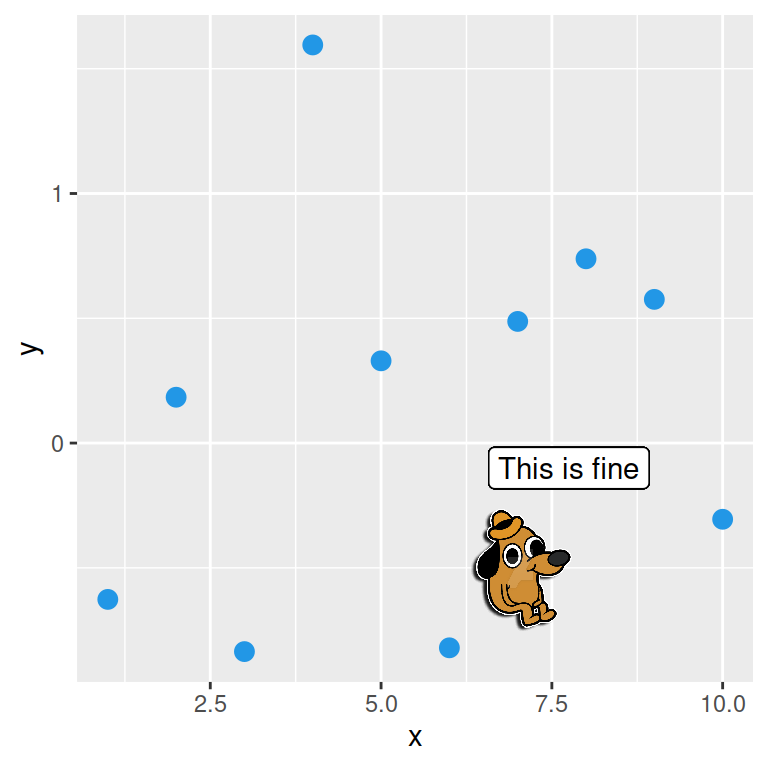``````# install.packages("remotes")
# remotes::install_github("R-CoderDotCom/ggdogs@main")
library(ggdogs)
# install.packages("ggplot2")
library(ggplot2)

# Sample data
set.seed(1)
df <- data.frame(x = 1:10, y = rnorm(10))

# Plot
ggplot(df, aes(x = x, y = y)) +
geom_point(size = 3, color = 4) +
geom_dog(aes(x = 7, y = -0.5), dog = "thisisfine", size = 5) +
geom_label(aes(x = 7.75, y = -0.1, label = "This is fine"))``````

Note that if your data frame contains a column with the name of a dog corresponding to each point you can pass that column to the `dog` argument to `aes`, so each point will have the corresponding dog.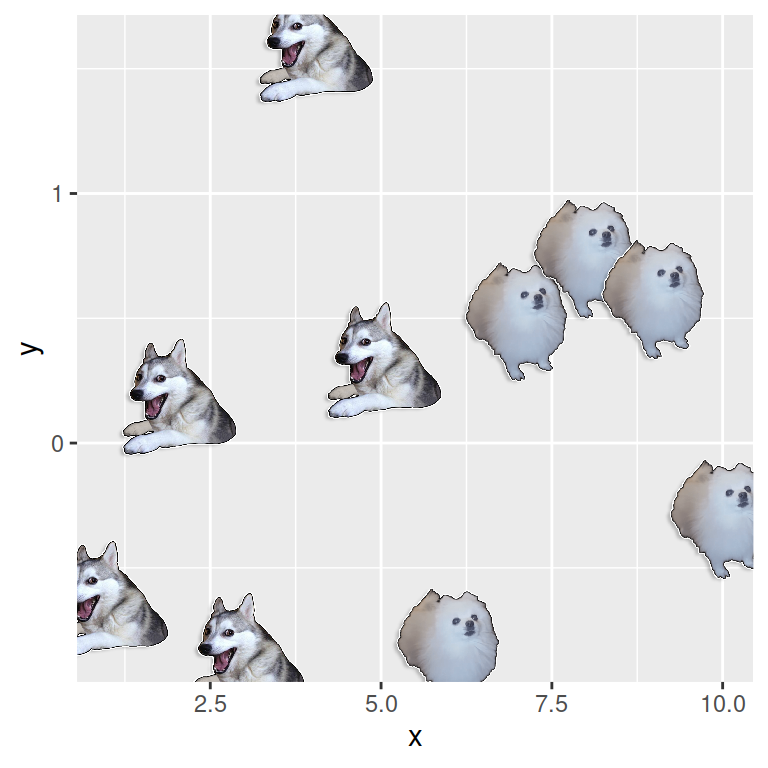``````# install.packages("remotes")
# remotes::install_github("R-CoderDotCom/ggdogs@main")
library(ggdogs)
# install.packages("ggplot2")
library(ggplot2)

# Sample data
set.seed(1)
df <- data.frame(x = 1:10, y = rnorm(10),
dog = c(rep("husky", 5),
rep("gabe", 5)))

# Plot
ggplot(df, aes(x = x, y = y, dog = dog)) +
geom_dog(size = 5) ``````

Finally, here is another cool example made with `ggdogs` and some Doge dogs: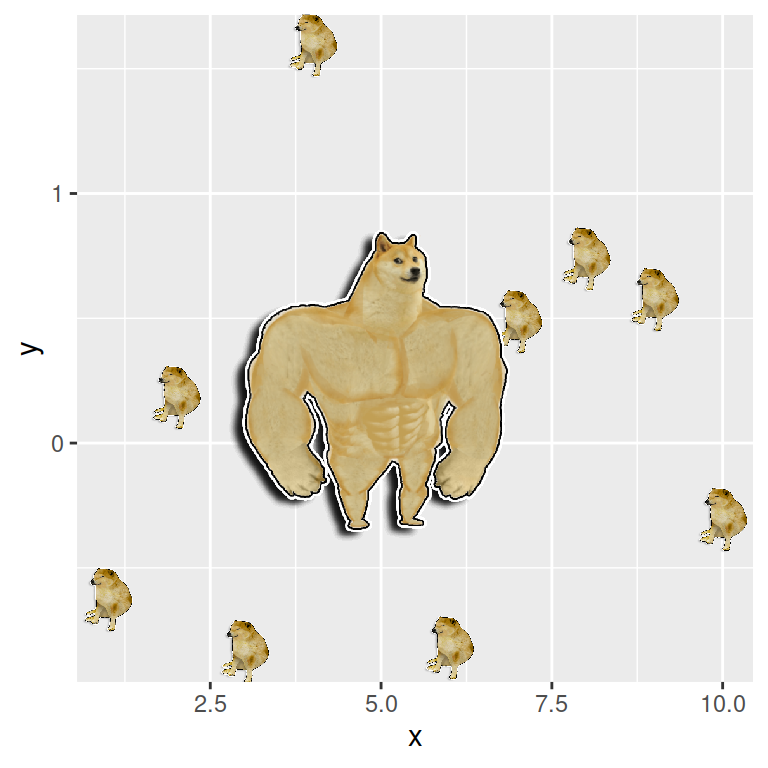``````# install.packages("remotes")
# remotes::install_github("R-CoderDotCom/ggdogs@main")
library(ggdogs)
# install.packages("ggplot2")
library(ggplot2)

# Sample data
set.seed(1)
df <- data.frame(x = 1:10, y = rnorm(10),
dog = c(rep("husky", 5),
rep("gabe", 5)))

# Plot
ggplot(df, aes(x = x, y = y)) +
geom_dog(size = 2.5) +
geom_dog(aes(x = 5, y = 0.25, dog = "doge_strong"), size = 12) ``````